# Practice Quiz in DC Biasing – FET Part 1

(Last Updated On: February 3, 2020)

This is the Online Practice Quiz in DC Biasing – FETs Part 1 from the book, Electronic Devices and Circuit Theory 10th Edition by Robert L. Boylestad. If you are looking for a reviewer in Electronics Engineering this will definitely help. I can assure you that this will be a great help in reviewing the book in preparation for your Board Exam. Make sure to familiarize each and every questions to increase the chance of passing the ECE Board Exam.

### Start Part I of the Online Practice Quiz

#### Quiz in DC Biasing – FETs

Question 1. Calculate VDS'.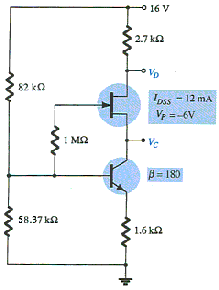A. 0 V

B. 6 V

C. 16 V

D. 11 V

Question 2. On the universal JFET bias curve, the vertical scale labelled _____ can, in itself, be used to find the solution to _____ configurations.

A. m, fixed-bias

B. M, fixed-bias

C. M, voltage-bias

D. m, voltage-bias

Question 3. Which of the following is (are) true of a self-bias configuration compared to a fixed-bias configuration?

A. One of the dc supplies is eliminated.

B. A resistor RS is added.

C. VGS is a function of the output current ID.

D. All of these

Question 4. For what value of RD is the voltage across VDS zero?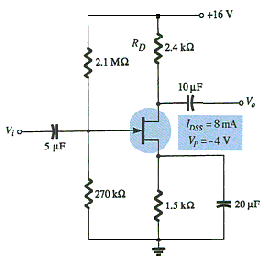A. 2.400 kΩ

B. 5.167 kΩ

C. 6.167 kΩ

D. 6.670 kΩ

Question 5. For the FET, the relationship between the input and output quantities is _____ due to the _____ term in Shockley's equation.

A. nonlinear, cubed

B. linear, proportional

C. nonlinear, squared

D. None of these

Question 6. At what value of RS does the circuit switch from depletion mode to enhancement mode?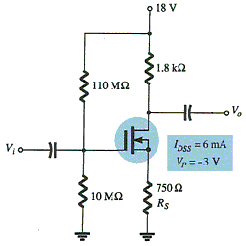A. 250 Ω

B. 500 Ω

C. 10 MΩ

D. None of these

Question 7. Seldom are current levels measured since such maneuvers require disturbing the network structure to insert the meter.

A. True

B. False

Question 8. Which of the following represents the voltage level of VGS in a self-bias configuration?

A. VG

B. VGS(off)

C. VS

D. VP

Question 9. Calculate the value of VDS.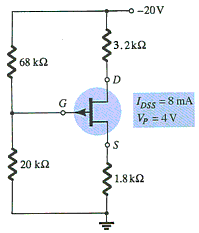A. –3 V

B. 3 V

C. –4 V

D. 4 V

Question 10. For what value of R2 is VGSQ equal to 1 V?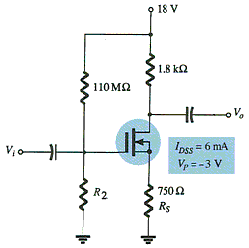A. 10 MΩ

B. 100 MΩ

C. 110 MΩ

D. 220 MΩ

### More Practice Quiz in DC Biasing – FETs

Practice Quiz Part 1

Practice Quiz Part 2

Practice Quiz Part 3

Practice Quiz Part 4

Practice Quiz Part 5

Practice Quiz Part 6

### See: Complete List of Practice Quizzes

Note: After taking this particular quiz, you can proceed to check all the topics.

Please do Subscribe on YouTube!

P inoyBIX educates thousands of reviewers and students a day in preparation for their board examinations. Also provides professionals with materials for their lectures and practice exams. Help me go forward with the same spirit.

“Will you subscribe today via YOUTUBE?”

Subscribe